## Interpret Machine Learning Models

This topic introduces Statistics and Machine Learning Toolbox™ features for model interpretation and shows how to interpret a machine learning model (classification and regression).

A machine learning model is often referred to as a "black box" model because it can be difficult to understand how the model makes predictions. Interpretability tools help you overcome this aspect of machine learning algorithms and reveal how predictors contribute (or do not contribute) to predictions. Also, you can validate whether the model uses the correct evidence for its predictions, and find model biases that are not immediately apparent.

### Features for Model Interpretation

Use `lime`, `shapley`, and `plotPartialDependence` to explain the contribution of individual predictors to the predictions of a trained classification or regression model.

• `lime` — Local interpretable model-agnostic explanations (LIME ) interpret a prediction for a query point by fitting a simple interpretable model for the query point. The simple model acts as an approximation for the trained model and explains model predictions around the query point. The simple model can be either a linear model or a decision tree model. You can use the estimated coefficients of a linear model or the estimated predictor importance of a decision tree model to explain the contribution of individual predictors to the prediction for the query point. For more details, see LIME.

• `shapley` — The Shapley value  of a predictor for a query point explains the deviation of the prediction (response for regression or class scores for classification) for the query point from the average prediction, due to the predictor. For a query point, the sum of the Shapley values for all features corresponds to the total deviation of the prediction from the average. For more details, see Shapley Values for Machine Learning Model.

• `plotPartialDependence` and `partialDependence` — A partial dependence plot (PDP ) shows the relationships between a predictor (or a pair of predictors) and the prediction (response for regression or class scores for classification) in the trained model. The partial dependence on the selected predictor is defined by the averaged prediction obtained by marginalizing out the effect of the other variables. Therefore, the partial dependence is a function of the selected predictor that shows the average effect of the selected predictor over the data set. You can also create a set of individual conditional expectation (ICE ) plots for each observation, showing the effect of the selected predictor on a single observation. For more details, see More About on the `plotPartialDependence` reference page.

Some machine learning models support embedded type feature selection, where the model learns predictor importance as part of the model learning process. You can use the estimated predictor importance to explain model predictions. For example:

For a list of machine learning models that support embedded type feature selection, see Embedded Type Feature Selection.

Use Statistics and Machine Learning Toolbox features for three levels of model interpretation: local, cohort, and global.

LevelObjectiveUse CaseStatistics and Machine Learning Toolbox Feature
Local interpretationExplain a prediction for a single query point.
• Identify important predictors for an individual prediction.

• Examine a counterintuitive prediction.

Use `lime` and `shapley` for a specified query point.
Cohort interpretationExplain how a trained model makes predictions for a subset of the entire data set.Validate predictions for a particular group of samples.
• Use `lime` and `shapley` for multiple query points. After creating a `lime` or `shapley` object, you can call the object function `fit` multiple times to interpret predictions for other query points.

• Pass a subset of data when you call `lime`, `shapley`, and `plotPartialDependence`. The features interpret the trained model using the specified subset instead of the entire training data set.

Global interpretationExplain how a trained model makes predictions for the entire data set.
• Demonstrate how a trained model works.

• Compare different models.

• Use `plotPartialDependence` to create PDPs and ICE plots for the predictors of interest.

• Find important predictors from a trained model that supports Embedded Type Feature Selection.

### Interpret Classification Model

This example trains an ensemble of bagged decision trees using the random forest algorithm, and interprets the trained model using interpretability features. Use the object functions (`oobPermutedPredictorImportance` and `predictorImportance`) of the trained model to find important predictors in the model. Also, use `lime` and `shapley` to interpret the predictions for specified query points. Then use `plotPartialDependence` to create a plot that shows the relationships between an important predictor and predicted classification scores.

Train Classification Ensemble Model

Load the `CreditRating_Historical` data set. The data set contains customer IDs and their financial ratios, industry labels, and credit ratings.

`tbl = readtable('CreditRating_Historical.dat');`

Display the first three rows of the table.

`head(tbl,3)`
```ans=3×8 table ID WC_TA RE_TA EBIT_TA MVE_BVTD S_TA Industry Rating _____ _____ _____ _______ ________ _____ ________ ______ 62394 0.013 0.104 0.036 0.447 0.142 3 {'BB'} 48608 0.232 0.335 0.062 1.969 0.281 8 {'A' } 42444 0.311 0.367 0.074 1.935 0.366 1 {'A' } ```

Create a table of predictor variables by removing the columns containing customer IDs and ratings from `tbl`.

`tblX = removevars(tbl,["ID","Rating"]);`

Train an ensemble of bagged decision trees by using the `fitcensemble` function and specifying the ensemble aggregation method as random forest (`'Bag'`). For reproducibility of the random forest algorithm, specify the `'Reproducible'` name-value argument as `true` for tree learners. Also, specify the class names to set the order of the classes in the trained model.

```rng('default') % For reproducibility t = templateTree('Reproducible',true); blackbox = fitcensemble(tblX,tbl.Rating, ... 'Method','Bag','Learners',t, ... 'CategoricalPredictors','Industry', ... 'ClassNames',{'AAA' 'AA' 'A' 'BBB' 'BB' 'B' 'CCC'});```

`blackbox` is a `ClassificationBaggedEnsemble` model.

Use Model-Specific Interpretability Features

`ClassificationBaggedEnsemble` supports two object functions, `oobPermutedPredictorImportance` and `predictorImportance`, which find important predictors in the trained model.

Estimate out-of-bag predictor importance by using the `oobPermutedPredictorImportance` function. The function randomly permutes out-of-bag data across one predictor at a time, and estimates the increase in the out-of-bag error due to this permutation. The larger the increase, the more important the feature.

`Imp1 = oobPermutedPredictorImportance(blackbox);`

Estimate predictor importance by using the `predictorImportance` function. The function estimates predictor importance by summing changes in the node risk due to splits on each predictor and dividing the sum by the number of branch nodes.

`Imp2 = predictorImportance(blackbox);`

Create a table containing the predictor importance estimates, and use the table to create horizontal bar graphs. To display an existing underscore in any predictor name, change the `TickLabelInterpreter` value of the axes to `'none'`.

```table_Imp = table(Imp1',Imp2', ... 'VariableNames',{'Out-of-Bag Permuted Predictor Importance','Predictor Importance'}, ... 'RowNames',blackbox.PredictorNames); tiledlayout(1,2) ax1 = nexttile; table_Imp1 = sortrows(table_Imp,'Out-of-Bag Permuted Predictor Importance'); barh(categorical(table_Imp1.Row,table_Imp1.Row),table_Imp1.('Out-of-Bag Permuted Predictor Importance')) xlabel('Out-of-Bag Permuted Predictor Importance') ylabel('Predictor') ax2 = nexttile; table_Imp2 = sortrows(table_Imp,'Predictor Importance'); barh(categorical(table_Imp2.Row,table_Imp2.Row),table_Imp2.('Predictor Importance')) xlabel('Predictor Importance') ax1.TickLabelInterpreter = 'none'; ax2.TickLabelInterpreter = 'none';```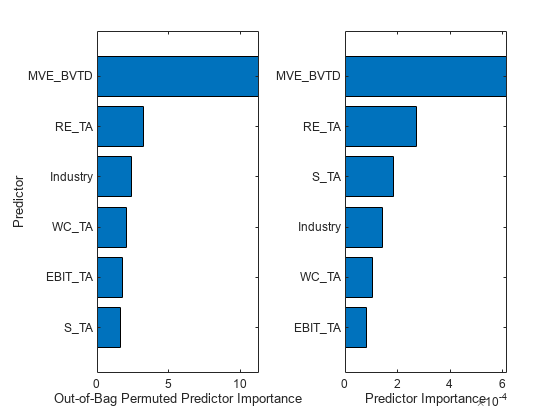Both object functions identify `MVE_BVTD` and `RE_TA` as the two most important predictors.

Specify Query Point

Find the observations whose `Rating` is `'AAA'` and choose four query points among them.

```tblX_AAA = tblX(strcmp(tbl.Rating,'AAA'),:); queryPoint = datasample(tblX_AAA,4,'Replace',false)```
```queryPoint=4×6 table WC_TA RE_TA EBIT_TA MVE_BVTD S_TA Industry _____ _____ _______ ________ _____ ________ 0.331 0.531 0.077 7.116 0.522 12 0.26 0.515 0.065 3.394 0.515 1 0.121 0.413 0.057 3.647 0.466 12 0.617 0.766 0.126 4.442 0.483 9 ```

Use LIME with Linear Simple Models

Explain the predictions for the query points using `lime` with linear simple models. `lime` generates a synthetic data set and fits a simple model to the synthetic data set.

Create a `lime` object using `tblX_AAA` so that `lime` generates a synthetic data set using only the observations whose `Rating` is `'AAA'`, not the entire data set.

`explainer_lime = lime(blackbox,tblX_AAA);`

The default value of 'DataLocality' for `lime` is `'global'`, which implies that, by default, `lime` generates a global synthetic data set and uses it for any query points. `lime` uses different observation weights so that weight values are more focused on the observations near the query point. Therefore, you can interpret each simple model as an approximation of the trained model for a specific query point.

Fit simple models for the four query points by using the object function `fit`. Specify the third input (the number of important predictors to use in the simple model) as 6 to use all six predictors.

```explainer_lime1 = fit(explainer_lime,queryPoint(1,:),6); explainer_lime2 = fit(explainer_lime,queryPoint(2,:),6); explainer_lime3 = fit(explainer_lime,queryPoint(3,:),6); explainer_lime4 = fit(explainer_lime,queryPoint(4,:),6);```

Plot the coefficients of the simple models by using the object function `plot`.

```tiledlayout(2,2) ax1 = nexttile; plot(explainer_lime1); ax2 = nexttile; plot(explainer_lime2); ax3 = nexttile; plot(explainer_lime3); ax4 = nexttile; plot(explainer_lime4); ax1.TickLabelInterpreter = 'none'; ax2.TickLabelInterpreter = 'none'; ax3.TickLabelInterpreter = 'none'; ax4.TickLabelInterpreter = 'none';```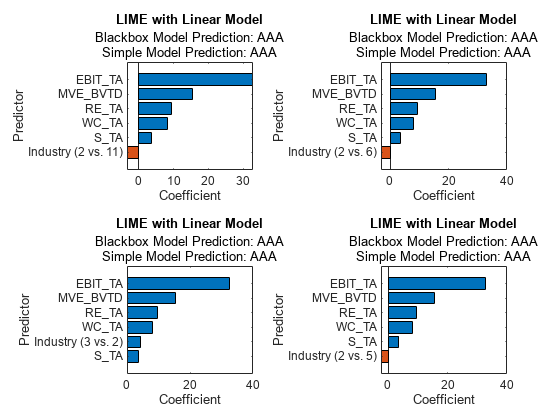All simple models identify `EBIT_TA`, `RE_TA`, and `MVE_BVTD` as the three most important predictors. The positive coefficients for the predictors suggest that increasing the predictor values leads to an increase in the predicted scores in the simple models.

For a categorical predictor, the `plot` function displays only the most important dummy variable of the categorical predictor. Therefore, each bar graph displays a different dummy variable.

Compute Shapley Values

The Shapley value of a predictor for a query point explains the deviation of the predicted score for the query point from the average score, due to the predictor. Create a `shapley` object using `tblX_AAA` so that `shapley` computes the expected contribution based on the samples for `'AAA'`.

`explainer_shapley = shapley(blackbox,tblX_AAA);`

Compute the Shapley values for the query points by using the object function `fit`.

```explainer_shapley1 = fit(explainer_shapley,queryPoint(1,:)); explainer_shapley2 = fit(explainer_shapley,queryPoint(2,:)); explainer_shapley3 = fit(explainer_shapley,queryPoint(3,:)); explainer_shapley4 = fit(explainer_shapley,queryPoint(4,:));```

Plot the Shapley values by using the object function `plot`.

```tiledlayout(2,2) ax1 = nexttile; plot(explainer_shapley1) ax2 = nexttile; plot(explainer_shapley2) ax3 = nexttile; plot(explainer_shapley3) ax4 = nexttile; plot(explainer_shapley4) ax1.TickLabelInterpreter = 'none'; ax2.TickLabelInterpreter = 'none'; ax3.TickLabelInterpreter = 'none'; ax4.TickLabelInterpreter = 'none';```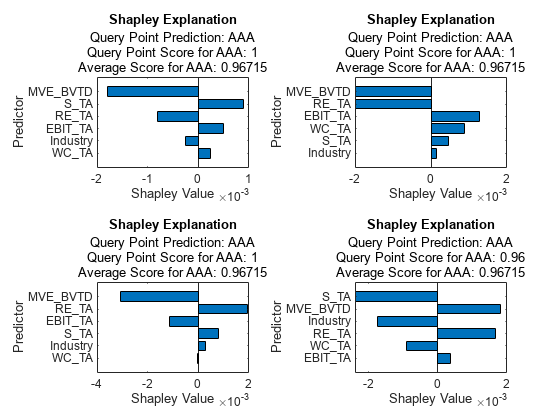`MVE_BVTD` and `RE_TA` are two of the three most important predictors for all four query points.

The Shapley values of `MVE_BVTD` are positive for the first and fourth query points, and negative for the second and third query points. The `MVE_BVTD` values are about 7 and 4 for the first and fourth query points, respectively, and the value for both the second and third query points is about 3.5. According to the Shapley values for the four query points, a large `MVE_BVTD` value leads to an increase in the predicted score, and a small `MVE_BVTD` value leads to a decrease in the predicted scores compared to the average. The results are consistent with the results from `lime`.

Create Partial Dependence Plot (PDP)

A PDP plot shows the averaged relationships between the predictor and the predicted score in the trained model. Create PDPs for `RE_TA` and `MVE_BVTD`, which the other interpretability tools identify as important predictors. Pass `tblx_AAA` to `plotPartialDependence` so that the function computes the expectation of the predicted scores using only the samples for `'AAA'`.

```figure plotPartialDependence(blackbox,'RE_TA','AAA',tblX_AAA)```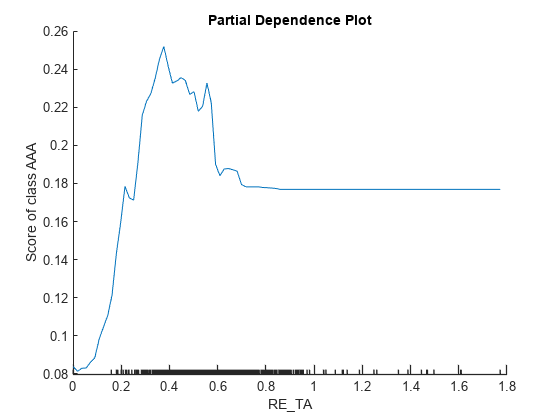`plotPartialDependence(blackbox,'MVE_BVTD','AAA',tblX_AAA)`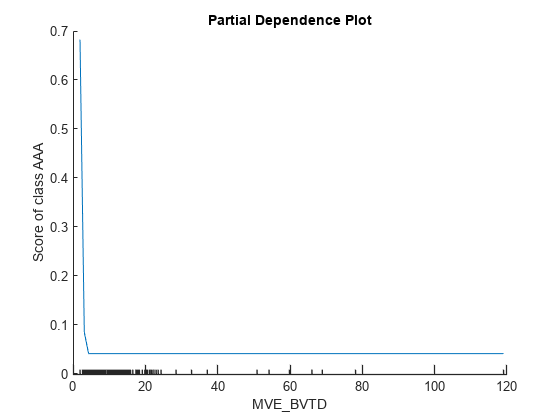The minor ticks in the `x`-axis represent the unique values of the predictor in `tbl_AAA`. The plot for `MVE_BVTD` shows that the predicted score is large when the `MVE_BVTD` value is small. The score value decreases as the `MVE_BVTD` value increases until it reaches about 5, and then the score value stays unchanged as the `MVE_BVTD` value increases. The dependency on `MVE_BVTD` in the subset `tbl_AAA` identified by `plotPartialDependence` is not consistent with the local contributions of `MVE_BVTD` at the four query points identified by `lime` and `shapley`.

### Interpret Regression Model

The model interpretation workflow for a regression problem is similar to the workflow for a classification problem, as demonstrated in the example Interpret Classification Model.

This example trains a Gaussian process regression (GPR) model and interprets the trained model using interpretability features. Use a kernel parameter of the GPR model to estimate predictor weights. Also, use `lime` and `shapley` to interpret the predictions for specified query points. Then use `plotPartialDependence` to create a plot that shows the relationships between an important predictor and predicted responses.

Train GPR Model

Load the `carbig` data set, which contains measurements of cars made in the 1970s and early 1980s.

`load carbig`

Create a table containing the predictor variables `Acceleration`, `Cylinders`, and so on

`tbl = table(Acceleration,Cylinders,Displacement,Horsepower,Model_Year,Weight);`

Train a GPR model of the response variable `MPG` by using the `fitrgp` function. Specify `'KernelFunction'` as `'ardsquaredexponential'` to use the squared exponential kernel with a separate length scale per predictor.

```blackbox = fitrgp(tbl,MPG,'ResponseName','MPG','CategoricalPredictors',[2 5], ... 'KernelFunction','ardsquaredexponential');```

`blackbox` is a `RegressionGP` model.

Use Model-Specific Interpretability Features

You can compute predictor weights (predictor importance) from the learned length scales of the kernel function used in the model. The length scales define how far apart a predictor can be for the response values to become uncorrelated. Find the normalized predictor weights by taking the exponential of the negative learned length scales.

```sigmaL = blackbox.KernelInformation.KernelParameters(1:end-1); % Learned length scales weights = exp(-sigmaL); % Predictor weights weights = weights/sum(weights); % Normalized predictor weights```

Create a table containing the normalized predictor weights, and use the table to create horizontal bar graphs. To display an existing underscore in any predictor name, change the `TickLabelInterpreter` value of the axes to `'none'`.

```tbl_weight = table(weights,'VariableNames',{'Predictor Weight'}, ... 'RowNames',blackbox.ExpandedPredictorNames); tbl_weight = sortrows(tbl_weight,'Predictor Weight'); b = barh(categorical(tbl_weight.Row,tbl_weight.Row),tbl_weight.('Predictor Weight')); b.Parent.TickLabelInterpreter = 'none'; xlabel('Predictor Weight') ylabel('Predictor')```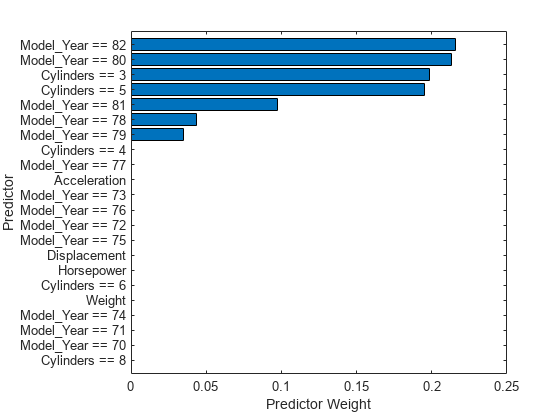The predictor weights indicate that multiple dummy variables for the categorical predictors `Model_Year` and `Cylinders` are important.

Specify Query Point

Find the observations whose `MPG` values are smaller than the 0.25 quantile of `MPG`. From the subset, choose four query points that do not include missing values.

```rng('default') % For reproducibility idx_subset = find(MPG < quantile(MPG,0.25)); tbl_subset = tbl(idx_subset,:); queryPoint = datasample(rmmissing(tbl_subset),4,'Replace',false)```
```queryPoint=4×6 table Acceleration Cylinders Displacement Horsepower Model_Year Weight ____________ _________ ____________ __________ __________ ______ 13.2 8 318 150 76 3940 14.9 8 302 130 77 4295 14 8 360 215 70 4615 13.7 8 318 145 77 4140 ```

Use LIME with Tree Simple Models

Explain the predictions for the query points using `lime` with decision tree simple models. `lime` generates a synthetic data set and fits a simple model to the synthetic data set.

Create a `lime` object using `tbl_subset` so that `lime` generates a synthetic data set using the subset instead of the entire data set. Specify `'SimpleModelType'` as `'tree'` to use a decision tree simple model.

`explainer_lime = lime(blackbox,tbl_subset,'SimpleModelType','tree');`

The default value of 'DataLocality' for `lime` is `'global'`, which implies that, by default, `lime` generates a global synthetic data set and uses it for any query points. `lime` uses different observation weights so that weight values are more focused on the observations near the query point. Therefore, you can interpret each simple model as an approximation of the trained model for a specific query point.

Fit simple models for the four query points by using the object function `fit`. Specify the third input (the number of important predictors to use in the simple model) as 6. With this setting, the software specifies the maximum number of decision splits (or branch nodes) as 6 so that the fitted decision tree uses at most all predictors.

```explainer_lime1 = fit(explainer_lime,queryPoint(1,:),6); explainer_lime2 = fit(explainer_lime,queryPoint(2,:),6); explainer_lime3 = fit(explainer_lime,queryPoint(3,:),6); explainer_lime4 = fit(explainer_lime,queryPoint(4,:),6);```

Plot the predictor importance by using the object function `plot`.

```tiledlayout(2,2) ax1 = nexttile; plot(explainer_lime1); ax2 = nexttile; plot(explainer_lime2); ax3 = nexttile; plot(explainer_lime3); ax4 = nexttile; plot(explainer_lime4); ax1.TickLabelInterpreter = 'none'; ax2.TickLabelInterpreter = 'none'; ax3.TickLabelInterpreter = 'none'; ax4.TickLabelInterpreter = 'none';```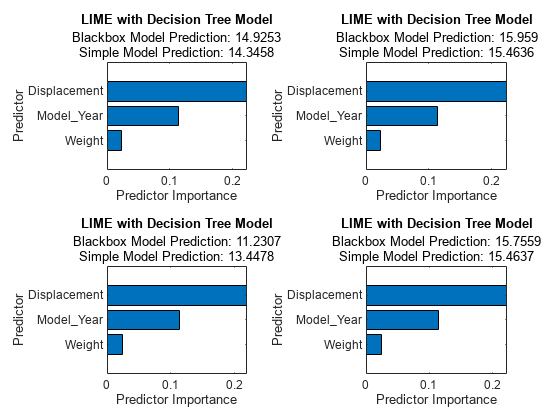All simple models identify `Displacement`, `Model_Year`, and `Weight` as important predictors.

Compute Shapley Values

The Shapley value of a predictor for a query point explains the deviation of the predicted response for the query point from the average response, due to the predictor. Create a `shapley` object for the model `blackbox` using `tbl_subset` so that `shapley` computes the expected contribution based on the observations in `tbl_subset`.

`explainer_shapley = shapley(blackbox,tbl_subset);`

Compute the Shapley values for the query points by using the object function `fit`.

```explainer_shapley1 = fit(explainer_shapley,queryPoint(1,:)); explainer_shapley2 = fit(explainer_shapley,queryPoint(2,:)); explainer_shapley3 = fit(explainer_shapley,queryPoint(3,:)); explainer_shapley4 = fit(explainer_shapley,queryPoint(4,:));```

Plot the Shapley values by using the object function `plot`.

```tiledlayout(2,2) ax1 = nexttile; plot(explainer_shapley1) ax2 = nexttile; plot(explainer_shapley2) ax3 = nexttile; plot(explainer_shapley3) ax4 = nexttile; plot(explainer_shapley4) ax1.TickLabelInterpreter = 'none'; ax2.TickLabelInterpreter = 'none'; ax3.TickLabelInterpreter = 'none'; ax4.TickLabelInterpreter = 'none';```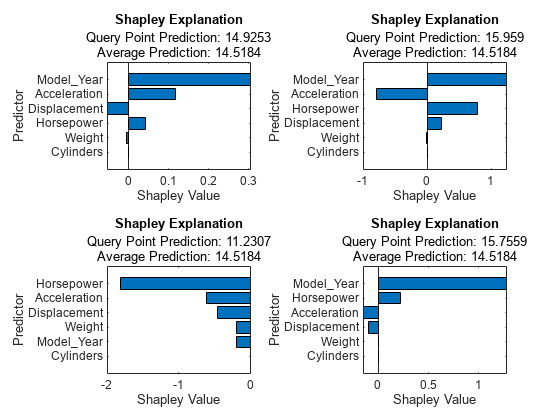`Model_Year` is the most important predictor for the first, second, and fourth query points, and the Shapley values of `Model_Year` are positive for the three query points. The `Model_Year` value is 76 or 77 for these three points, and the value for the third query point is 70. According to the Shapley values for the four query points, a small `Model_Year` value leads to a decrease in the predicted response, and a large `Model_Year` value leads to an increase in the predicted response compared to the average.

Create Partial Dependence Plot (PDP)

A PDP plot shows the averaged relationships between the predictor and the predicted response in the trained model. Create a PDP for `Model_Year`, which the other interpretability tools identify as an important predictor. Pass `tbl_subset` to `plotPartialDependence` so that the function computes the expectation of the predicted responses using only the samples in `tbl_subset`.

```figure plotPartialDependence(blackbox,'Model_Year',tbl_subset)```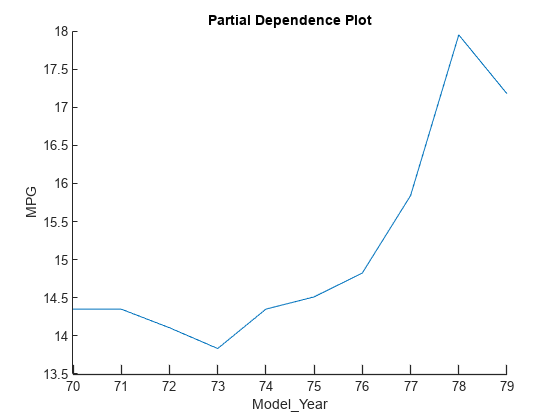The plot shows the same trend identified by the Shapley values for the four query points. The predicted response (`MPG`) value increases as the `Model_Year` value increases.

 Ribeiro, Marco Tulio, S. Singh, and C. Guestrin. "'Why Should I Trust You?': Explaining the Predictions of Any Classifier." In Proceedings of the 22nd ACM SIGKDD International Conference on Knowledge Discovery and Data Mining, 1135–44. San Francisco, California: ACM, 2016.

 Lundberg, Scott M., and S. Lee. "A Unified Approach to Interpreting Model Predictions." Advances in Neural Information Processing Systems 30 (2017): 4765–774.

 Aas, Kjersti, Martin. Jullum, and Anders Løland. "Explaining Individual Predictions When Features Are Dependent: More Accurate Approximations to Shapley Values." arXiv:1903.10464 (2019).

 Friedman, Jerome. H. “Greedy Function Approximation: A Gradient Boosting Machine.” The Annals of Statistics 29, no. 5 (2001): 1189-1232.

 Goldstein, Alex, Adam Kapelner, Justin Bleich, and Emil Pitkin. “Peeking Inside the Black Box: Visualizing Statistical Learning with Plots of Individual Conditional Expectation.” Journal of Computational and Graphical Statistics 24, no. 1 (January 2, 2015): 44–65.